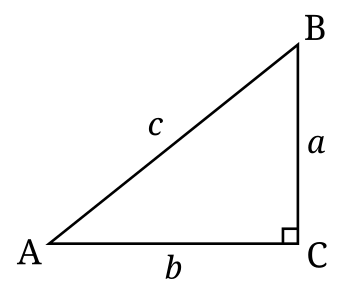# Hypotenuse facts for kids

Kids Encyclopedia Facts

The hypotenuse is the side opposite the 90 degrees angle in a right triangle. It is always the longest side.

For example:In this triangle, if angle C is 90 degrees, then the opposite side, "c", is the hypotenuse. The hypotenuse is important in trigonometry, where it is used to calculate many functions.

The length of the hypotenuse can be calculated if the other two sides are known, using Pythagoras' Theorem. In fact, the length of any side of a right triangle can be calculated if the other two sides are known, using this theorem.Hypotenuse Facts for Kids. Kiddle Encyclopedia.